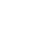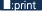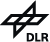Definition and conversion of volume mixing ratio Definition The volume mixing ratio, or simply mixing ratio, of a gas is defined as the ratio of the number density of the gas to the total number density of the atmosphere. In other words, ozone volume mixing ratio is the density of ozone divided by the density of all constituents in a unit volume (i.e. number of molecules per unit volume). Therefore, an ozone mixing ratio of 10-6 means that the number density of ozone is 10-6 times the total number density of air in a unit volume. Following the standard convention for the Earth's troposphere and stratosphere, this mixing ratio equals 1 ppmv (parts per million by volume). Conversion of volume mixing ratio Below conversions of ozone volume mixing ratio (vmr) into common units are listed. The volume mixing ratio is given in ppmv (parts per million, 10-6), pressure in hPa and temperature in K. Ozone partial pressure pO3 [nbar]: pO3 = vmrO3 * pressure Ozone density ρO3[μg/m3]: ρO3 = vmrO3 * 577.3 * pressure / temperature Ozone mass mixing ratio mO3 [μg/g]: mO3 = vmrO3 * 1.66 Ozone number density NO3 [molecules/cm3]: NO3 = vmrO3 * pressure / (1.38 10-19 * temperature) Conversion into volume mixing ratio Below conversions of common units into ozone volume mixing ratio (vmr) are outlined. The volume mixing ratio is given in ppmv, pressure in hPa and temperature in K. Ozone partial pressure pO3 [nbar]: vmrO3 = pO3 / pressure Ozone density ρO3 [μg/m3]: vmrO3 = ρO3 * 1.7322 * 10-3 * temperature / pressure Ozone mass mixing ratio mO3 [μg/g]: vmrO3 = mO3 * 0.602 Ozone number density NO3 [molecules/cm3]: vmrO3 = NO3 * 1.38 10-19 * temperature / pressure Thilo Erbertseder, Frank Baier, last modified: October 2005